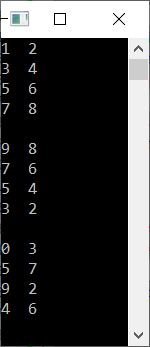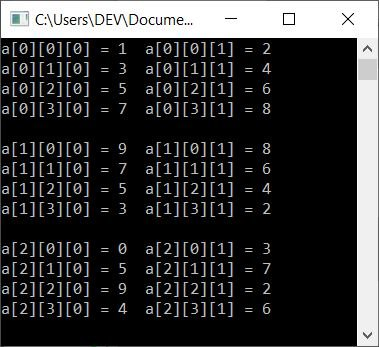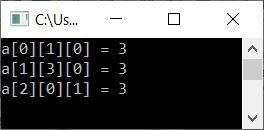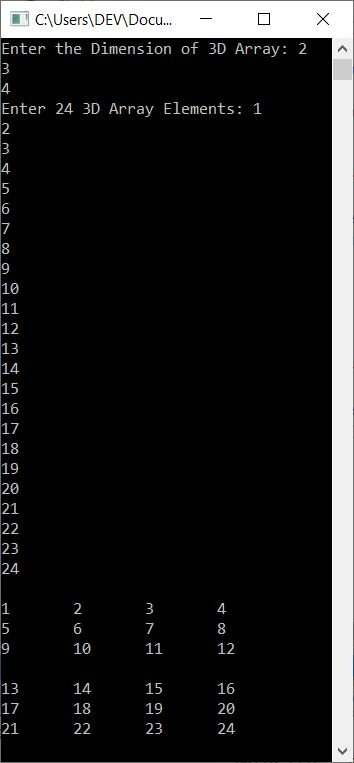# C++ Three-Dimensional Array Programs

In this article, you will learn how to implement a three-dimensional (3D) array in C++ and get the code to do so. Here is the list of programs available in this article:

Note: A three-dimensional (3D) array comes under the category of a multi-dimensional array. A multi-dimensional array is an array of arrays.

Note: A three-dimensional (3D) array is a collection of two-dimensional (2D) arrays.

Note: In a 3D array, there are three dimensions (subscripts). The first shows block size, the second shows row size, and the third shows column size. The dimensions of a 2D array are represented by the row and column sizes. The block size, on the other hand, indicates the number of 2D arrays. For example, if a 3D array's dimension is 3*4*2, it is a 3 2D array with 4 * 2 dimensions. Furthermore, it refers to a three-by-two-dimensional array with four rows and two columns.

## 3D Array Program in C++

This program initializes elements in a three-dimensional array named threeDimArr[][][] of size 3*4*2. After all 24 elements get initialized, we print the 3D array back on the output screen as shown in the program given below:

```#include<iostream>
using namespace std;
int main()
{
int i, j, k;
int threeDimArr = {
{ {1, 2}, {3, 4}, {5, 6}, {7, 8} },
{ {9, 8}, {7, 6}, {5, 4}, {3, 2} },
{ {0, 3}, {5, 7}, {9, 2}, {4, 6} }
};
for(i=0; i<3; i++)
{
for(j=0; j<4; j++)
{
for(k=0; k<2; k++)
cout<<threeDimArr[i][j][k]<<"  ";
cout<<endl;
}
cout<<endl;
}
cout<<endl;
return 0;
}```

This program was built and runs under the Code::Blocks IDE. Here is its sample output:Note: As you can see, there are three 2-dimensional arrays of size 4*2. That is, each two-dimensional array contains 4 rows and 2 columns.

To print a 3D array, you have to use three for loops. The third for loop (the innermost loop) forms a 1D array, the second for loop forms a 2D array, and the third for loop (the outermost loop) forms a 3D array.

In other words, the block size is the outermost (first) dimension of the loop, the row size of the 2D array is the second, and the column size of the 2D array is the third.

### Print three-dimensional array with their indexes in C++

This program is similar to the previous program. In addition to the job done by the previous program, this program prints all elements of a 3D array along with indexes. So this program also shows how elements get stored in a 3D array:

```#include<iostream>
using namespace std;
int main()
{
int i, j, k;
int a = {
{ {1, 2}, {3, 4}, {5, 6}, {7, 8} },
{ {9, 8}, {7, 6}, {5, 4}, {3, 2} },
{ {0, 3}, {5, 7}, {9, 2}, {4, 6} }
};
for(i=0; i<3; i++)
{
for(j=0; j<4; j++)
{
for(k=0; k<2; k++)
cout<<"a["<<i<<"]["<<j<<"]["<<k<<"] = "<<a[i][j][k]<<"  ";
cout<<endl;
}
cout<<endl;
}
cout<<endl;
return 0;
}```

The snapshot given below shows the sample output produced by this C++ program on a three-dimensional array:### Access and print elements of a three-dimensional array

Now this C++ program shows how the elements of a 3D array get accessed. In this program, we've accessed three elements of a 3D array using its index.

```#include<iostream>
using namespace std;
int main()
{
int i, j, k;
int a = {
{ {1, 2}, {3, 4}, {5, 6}, {7, 8} },
{ {9, 8}, {7, 6}, {5, 4}, {3, 2} },
{ {0, 3}, {5, 7}, {9, 2}, {4, 6} }
};
cout<<"a = "<<a<<endl;
cout<<"a = "<<a<<endl;
cout<<"a = "<<a<<endl;
cout<<endl;
return 0;
}```

Here is its sample output:Note: In the above program, a 1D array of two elements is constructed first. Then four such 1D arrays are placed one below the other to give a 2D array containing four rows. Then, three such 2D arrays are placed one behind the other to yield a 3D array containing three 2D arrays.

### Receive 3D array elements from the user

This program allows the user to enter the dimension and then elements for a 3D array. Based on user inputs, we've printed the 3D array back on the output screen:

```#include<iostream>
using namespace std;
int main()
{
int i, j, k, a;
int one, two, three;
cout<<"Enter the Dimension of 3D Array: ";
cin>>one>>two>>three;
cout<<"Enter "<<one*two*three<<" 3D Array Elements: ";
for(i=0; i<one; i++)
{
for(j=0; j<two; j++)
{
for(k=0; k<three; k++)
cin>>a[i][j][k];
}
}
cout<<endl;
for(i=0; i<one; i++)
{
for(j=0; j<two; j++)
{
for(k=0; k<three; k++)
cout<<a[i][j][k]<<"  ";
cout<<endl;
}
cout<<endl;
}
cout<<endl;
return 0;
}```

Here is its sample run with user input, 2, 3, 4 as dimensions, and 1, 2, 3, 4, 5, 6, 7, 8, 9, 10, 11, 12, 13, 14, 15, 16, 17, 18, 19, 20, 21, 22, 23, 24 as 24 3D array elements:#### The same program in different languages

C++ Quiz

« Previous Program Next Program »